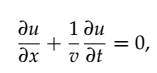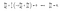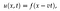# Travelling wave solutions to partial differential equations

In the world of mathematics, partial differential equations (abbreviated as PDEs) are used for modelling natural phenomena, from very basic transport and diffusion processes (e.g. kinetic transport of gas molecules inside a closed system), to more complicated physical processes (such as fluid dynamics around complicated geometries). These equations are often unsolvable analytically, and so we often employ powerful computers and specialized software in order to find approximate solutions. However, there are special cases in which analytic solutions to these PDEs do exist, and these special analytic solutions can tell us a lot about the behaviour of such a physical system, for example how would a wave propagate along the surface of a shallow water pond before breaking? These solutions also serve as a reference point from which we can test the accuracy of the numerical methods we develop for more complicated cases.

One such type of analytic solution is often called a “Travelling wave solution” because the resulting function behaves like a wave that propagates at a constant speed without changing its shape. To illustrate how one can arrive at such a solution, consider a simple one-dimensional advection equationEquation 1: a 1D advection equation

The idea is now to introduce a certain set of variable transformations such that the two partial derivatives can be reduced to just one. In this case, we can try

Then, using the chain rule of partial derivatives, we can make the following transformations

which upon substitution in to Eq(1) yieldsEquation 2: advection equation in a transformed coordinate system

What this means is that we have essentially moved our solution u(x,t) into a new coordinate system which propagates at the same speed v as the solution u(x,t) itself, hence why one of the partial derivatives cancels out. This newly transformed equation is in fact an ordinary differential equation (ODE) which can be solved by integrating both sides of Eq(2), leading to

for some arbitrary constant c. Next, suppose we have the initial condition

then it follows that our arbitrary constant is in fact

and substituting back for

yields the solution in the original coordinate systemEquation 3: travelling wave solution to the advection equation

What this effectively means is that any function f(x-vt) satisfies Eq(1), so we could for example write something like

Any of these will actually be a valid solution to the PDE.

So how do we know if a PDE has a travelling wave solution? If some coordinate transformation exists such that the PDE can be reduced to a single ODE (e.g. we get all of the partial derivatives cancelling out except for one of them), and that ODE has an exact solution, then we say that the PDE has a travelling wave solution, provided it is expressible as some function of the term x-vt (if you think of v as the speed, then this is the same as shifting the solution by a distance vt with each second that passes).

The amazing thing about travelling wave solutions is that they are not necessarily restricted to linear PDEs. There exist nonlinear PDEs also which possess exact travelling wave solutions, like the Korteweg-de Vries equation (KdV equation), which models shallow water waves. We will look at some of these examples some time later.

Oscar is a physicist, educator and STEM enthusiast. He is currently finishing a PhD in Theoretical Physics with a focus on photonics and stochastic dynamics.

## More from Oscar Nieves

Oscar is a physicist, educator and STEM enthusiast. He is currently finishing a PhD in Theoretical Physics with a focus on photonics and stochastic dynamics.